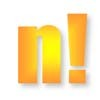Search by Topic

Filter by: Content type:
Age range:
Challenge level:

There are 7 NRICH Mathematical resources connected to Prime numbers, you may find related items under Numbers and the Number System.

Broad Topics > Numbers and the Number System > Prime numbersFactors and Multiples Game

Age 7 to 16 Challenge Level:

A game in which players take it in turns to choose a number. Can you block your opponent?Why 24?

Age 14 to 16 Challenge Level:

Take any prime number greater than 3 , square it and subtract one. Working on the building blocks will help you to explain what is special about your results.Prime Counter

Age 16 to 18 Challenge Level:

A short challenge concerning prime numbers.Prime Sequences

Age 16 to 18 Challenge Level:

This group tasks allows you to search for arithmetic progressions in the prime numbers. How many of the challenges will you discover for yourself?An Introduction to Proof by Contradiction

Age 14 to 18

An introduction to proof by contradiction, a powerful method of mathematical proof.Different by One

Age 14 to 16 Challenge Level:

Make a line of green and a line of yellow rods so that the lines differ in length by one (a white rod)Factorial Fun

Age 16 to 18 Challenge Level:

How many divisors does factorial n (n!) have?